Next: Magnetism Up: Electric Current Previous: Example 7.3: Kirchhoff's rules

## Example 7.4: Energy in DC circuits

Question: A 150W light bulb is connected to a 120V line. What is the current drawn from the line? What is the resistance of the light bulb whilst it is burning? How much energy is consumed if the light is kept on for 6 hours? What is the cost of this energy at 8 cents/kWh?

Answer: Since power is equal to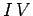, it follows thatFrom Ohm's law, the resistance of the light bulb is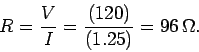The energy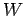consumed is the product of the power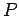(the energy consumed per unit time) and the time periodfor which the light is on, so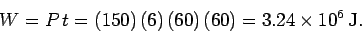Since,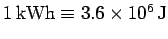, it follows that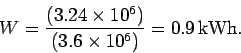The costof the electricity is product of the number of kilowatthours used and the cost per kilowatthour, so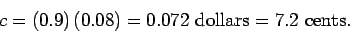Next: Magnetism Up: Electric Current Previous: Example 7.3: Kirchhoff's rules
Richard Fitzpatrick 2007-07-14